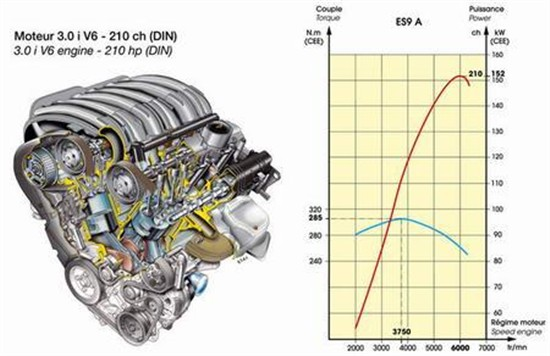This is a GOOD read. If you want to understand Torque and Horsepower, read it.

In short:
Torque and work measurements tell us how much has been accomplished, but provides no clue how fast a given amount of work (or torque) is done. That’s the job of power, an expression of the rate or speed at which work is performed. The more power that is generated, the more work is done in a given time-period.

Torque Explained:
Torque is about how much the WORK is done.
Work = D (distance moved) x F (force applied)
For example:
You pushes (with a force of 20 pounds) a table to move forward 10 meters. Then the work done is: work = 10 meters * 20 pounds.
Engine’s torque is 320 NM, Newton*Meter (force*distance).
BUT the problem here is: Torque is only about how much work is done – 10 meters movement of table forwards with a power of 20 pounds. But it does NOT tell us about HOW FAST the work is done – how fast you move the table forwards.

Horsepower Explained:
Torque and work measurements tell us how much has been accomplished, but provides no clue how fast a given amount of work (or torque) is done. That’s the job of power, an expression of the rate or speed at which work is performed. The more power that is generated, the more work is done in a given time-period.

Suppose it takes a constant 100 lb-ft of torque to spin a nut onto a bolt one complete revolution. Your girlfriend takes 10 seconds to do this. You, being a real stud (pun intended), take only 5 seconds to perform the same task. You would be twice as powerful, because you performed the same work in half the time.

In the U.S. system, power is expressed as “horsepower” (hp). One hp is the amount of power it takes to perform 33,000 ft-lb of work in 1 minute.

ENGINE SPEE: RPM*1000功率曲线比较陡，这表明发动机的功率随着转速的提高而急剧上升，其峰顶对应的功率数值即为发动机技术参数中标注的“最大功率”。最大功率越大，汽车可能达到的最高车速也越高。The graph is for a 9-3SS Aero 2.0T MY2007.

The max power (224.2HP) is achieved at 5004 rpm, which is relatively early.
The max torque is 329.8Nm at 3005 rpm.